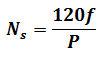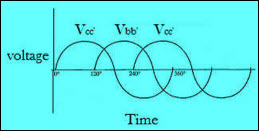# Synchronous Generator Working Principle

The electrical machine can be defined as a device that converts electrical energy into mechanical energy or mechanical energy into electrical energy. An electrical generator can be defined as an electrical machine that converts mechanical energy into electrical energy. An electrical generator typically consists of two parts; stator and rotor. There are various types of electrical generators such as direct current generators, alternating current generators, vehicular generators, human powered electrical generators, and so on. In this article, let us discuss about synchronous generator working principle.

## Synchronous Generator

The rotating and stationary parts of an electrical machine can be called as rotor and stator respectively. The rotor or stator of electrical machines acts as a power-producing component and is called as an armature. The electromagnets or permanent magnets mounted on the stator or rotor are used to provide magnetic field of an electrical machine. The generator in which permanent magnet is used instead of coil to provide excitation field is termed as permanent magnet synchronous generator or also simply called as synchronous generator.

### Construction of Synchronous Generator

In general, synchronous generator consists of two parts rotor and stator. The rotor part consists of field poles and stator part consists of armature conductors. The rotation of field poles in the presence of armature conductors induces an alternating voltage which results in electrical power generation.

The speed of field poles is synchronous speed and is given byWhere, ‘f’ indicates alternating current frequency and ‘P’ indicates number of poles.

### Synchronous Generator Working Principle

The principle of operation of synchronous generator is electromagnetic induction. If there exits a relative motion between the flux and conductors, then an emf is induced in the conductors. To understand the synchronous generator working principle, let us consider two opposite magnetic poles in between them a rectangular coil or turn is placed as shown in the below figure.

If the rectangular turn rotates in clockwise direction against axis a-b as shown in the below figure, then after completing 90 degrees rotation the conductor sides AB and CD comes in front of the S-pole and N-pole respectively. Thus, now we can say that the conductor tangential motion is perpendicular to magnetic flux lines from north to south pole.

So, here rate of flux cutting by the conductor is maximum and induces current in the conductor, the direction of the induced current can be determined using Fleming’s right hand rule. Thus, we can say that current will pass from A to B and from C to D. If the conductor is rotated in a clockwise direction for another 90 degrees, then it will come to a vertical position as shown in the below figure.